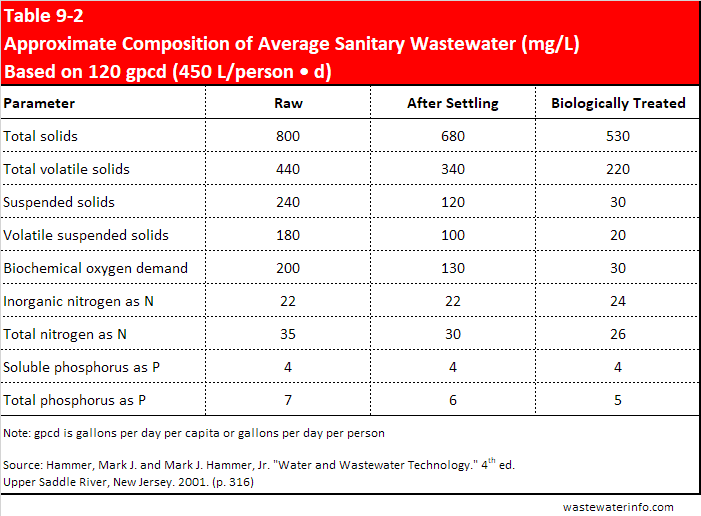# The Weight of Water

## The Unit of "8.34 pounds per gallon" Explained

In the United States a common unit of measurement for water is that it weighs 8.34 pounds per gallon. And the discussion that follows has to do with U.S. customary unit of measurements.

A comment was made to me recently that water does not weigh 8.34 pounds per gallon and that the value of 8.34 was the result of a completely unrelated (to the weight of water) derivation. I think I understood the confusion so I thought I would go to an excellent source and simply quote the following information from Hammer and Hammer. This should provide you with all you ever wanted to know, and more, about the use of 8.34 in water and wastewater treatment.Industrial Wastewater Discharge

Loadings on treatment units are often expressed in terms of pounds of BOD per day or pounds of solids per day, as well as quantity of flow per day. The relationship between the parameters of concentration and flow is based on the following conversion factors: 1.0 mg/L, which is the same as 1.0 part per million parts by weight, equals 8.34 lb/mil gal, since 1 gallon of water weighs 8.34 lb; and used less frequently, the value 62.4 lb/mil cu ft, since 1 cu ft of water weighs 62.4 lb. These relationships are defined by the following equations:

Equation 9-1Equation 9-2Where:

C = BOD, SS, or other constituent, milligrams per liter (mg/L)

Q = volume of wastewater, million gallons (mil gal) or million cubic feetCalculations in Example 9-1 show that 120 gal of the sanitary wastewater described in Table 9-2 (shown below) contain 0.20 lb of BOD and 0.24 lb of suspended solids.

Example 9-1

Sanitary wastewater from a residential community is 120 gpcd containing 200 mg/L BOD and 240 mg/L suspended solids. Compute the pounds of BOD per capita and pounds of suspended solids per capita.

Solution, using Equation 9-1Domestic Wastewater Composition

Source: Hammer, Mark J. and Mark J. Hammer, Jr. “Water and Wastewater Technology.” 4th ed. Prentice Hall: Upper Saddle River, New Jersey. 2001. (p. 316)

## Units of Measurement

In quoting from Hammer and Hammer in the section above the following statement was made: "The relationship between the parameters of concentration and flow is based on the following conversion factors: 1.0 mg/L, which is the same as 1.0 part per million parts by weight..." but ppm and mg/L are not always equal. Let me clarify when ppm and mg/L are equal to one another.

Measurements of chemical parameters are usually expressed in the physical unit of milligrams per liter (mg/L) or grams per cubic meter (g/m3). The concentration can also be expressed as parts per million (ppm), which is a mass-to-mass ratio. The relationship between mg/L and ppm is:For dilute systems, such as those encountered in natural waters and wastewater, in which one liter of sample weighs approximately one kilogram, the units of mg/L or g/m3 are interchangeable with ppm.

Source: Metcalf & Eddy. “Wastewater Engineering, Treatment and Reuse.” Fourth edition. McGraw Hill: New York. 2003. (p. 35)